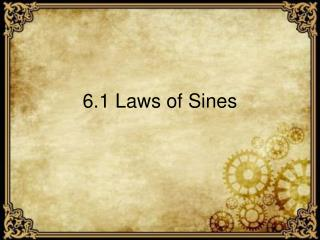Download Presentation6.1 Laws of Sines6.1 Laws of Sines - PowerPoint PPT Presentation

Download Presentation6.1 Laws of Sines
An Image/Link below is provided (as is) to download presentation

Download Policy: Content on the Website is provided to you AS IS for your information and personal use and may not be sold / licensed / shared on other websites without getting consent from its author. While downloading, if for some reason you are not able to download a presentation, the publisher may have deleted the file from their server.

- - - - - - - - - - - - - - - - - - - - - - - - - - - E N D - - - - - - - - - - - - - - - - - - - - - - - - - - -
Presentation Transcript

1. 6.1 Laws of Sines

2. The Laws of Sine can be used with Oblique triangle Oblique triangle is a triangle that contains no right angle.

3. The Laws of Sines

4. Using the Law of Sines Given: How do you find angle B?

5. Using the Law of Sines Given: How do you find side b?

6. Using the Law of Sines Given: How do you find side b?

7. Using the Law of Sines Given: How do you find side b?

8. Using the Law of Sines Given: How do you find side c?

9. Using the Law of Sines Given: How do you find side c?

10. The Ambiguous Case Look at this triangle. If we look at where angle A Is Acute

11. The Ambiguous Case Look at this triangle. If we look at If a = h, then there is one triangle

12. The Ambiguous Case Look at this triangle. If we look at If a < h, then there is no triangle

13. The Ambiguous Case Look at this triangle. If we look at If a > b, then there is one triangle

14. The Ambiguous Case Look at this triangle. If we look at If h< a <b, then there is two triangles

15. The Ambiguous Case Do you remember the Hinge Theorem from Geometry. Given two sides and one angle, two different triangles can be made. http://mrself.weebly.com/5-5-the-hinge-theorem.html

16. The Ambiguous Case Where Angle A is Obtuse. If a ≤ b, there is no triangle

17. The Ambiguous Case Where Angle A is Obtuse. If a > b, there is one triangle

18. Area of an Oblique triangle Using two sides and an Angle.

19. Homework Page 416 # 1, 7, 13, 19, 25, 31, 37, 43, 49

20. Homework Page 416 # 4, 10, 16, 22, 28, 34, 40, 46, 52